# Bihar Board Class 10 Science Solutions Chapter 12 Electricity

## BSEB Bihar Board Class 10 Science Solutions Chapter 12 Electricity

Bihar Board Class 10 Science Solutions Chapter 12 Electricity Textbook Questions and Answers, Additional Important Questions, Notes.

### Bihar Board Class 10 Science Chapter 12 Electricity InText Questions and Answers

Intext Questions (Page 200)

Question 1.
What does an electric circuit mean ?
Combination of electrical devices connected by conducting wires is called an electric circuit.

Question 2.
Define the unit of electric current.
The rate of flow of charge is called electric, current and its S.I. unit is ampere. One ampere current is defined as below :
“If one coulomb charge flows through a conductor per second, the strength of current is called one ampere.”

Question 3.
Calculate the number of electrons -constituting one coulomb of charge.
We know charge on one electron = 1.6x 10-19 C
Let the number of electrons constituting 1 coulomb charge = n.n = 6.25 x 1018 electrons

Intext Questions (Page 202)

Question 1.
Name a device that helps to maintain a potential difference across a conductor.
Cell is the device that helps to maintain a potential difference* across a conductor.Question 2.
What is meant by saying that the potential difference between two points is 1 V?
Potential difference of 1 volt means that the rate of doing work during transfer of per coulomb charge is 1 joule.

Question 3.
How much energy is given to each coulomb of charge passing through a 6V battery?
We know
W = V x Q
W = 6 x 1
W = 6 joules

Intext Questions (Page 209)

Question 1.
On what factors does the resistance of a conductor depend?
Resistance of a conductor depends on :
(i) Nature of material (resistivity – p).
(ii) Length of wire (l).
∴ R ∝ l
(iii) The cross-sectional area of the wire (A).
R = $$\frac {1}{A}$$ (A = πr2 or l x b)

Question 2.
Will current flow more easily a thick wire or a thin wire of the same material, when connected to the same source ? Why ?
The current will flow easily through the thick the wire because the resistance of thick wire is less than thin wire.
R ∝$$\frac {1}{A}$$

Question 3.
Let the resistance of an electrical component remains constant while the potential difference across the two ends of the component decreases to half of its former value. What change will occur in the current through it?
At constant temperature and resistance :
V ∝ IQuestion 4.
Why are coils of electric toasters and electric irons made of an alloy rather than a pure metal ?
Alloys have more resistance then constituent metals. So, they are heated up easily due to the heating effect of electric current.

Intext Questions (Page 213)

Question 1.
Draw a schematic diagram of a circuit consisting of a battery of three cells of 2 V each, a 5vΩ resistor, an 8 Ω resistor, 12 Ω resistor, and a plug key, all connected in series.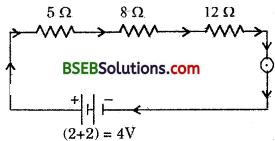Question 2.
Redraw the circuit of Ω. 1 putting in an ammeter to measure the current through the resistors and a voltmeter to measure the potential difference across the 12 Ω resistor. What would be the readings in the ammeter and the voltmeter ?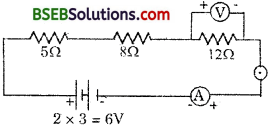Equivalent resistance = 5 + 8 + 12
R = 25 Ω
We know I = $$\frac {V}{R}$$
I = $$\frac {6}{25}$$
I = 0.24 ampere
PD. across 12 Ω resistor = IR
= 0.24 x 12
= 2.88 volts

Intext Questions (Page 216)

Question 1.
Judge the equivalent resistance when the following are connected in parallel (a) 1 Ω and 106 Ω (b) 1 Ω, 103 Ω and 106 Ω.Question 2.
An electric lamp of 100 Ω, a toaster of resistance 50 Ω, and a water filter of resistance 500 Ω are connected in parallel to a 220 V source. What is the resistance of an electric iron connected to the same source that takes as must current as all three appliances and what is the current through it?
The electric iron will have equivalent resistance to the same as 100 Ω, 50 Ω and 500 Ω resistors are in parallel.
Let it be R Ω.Question 3.
What are the advantages of connecting electrical devices in parallel with the battery instead of connecting them in series?
The equivalent resistance of resistances connected in parallel is less than even the smallest resistance connected in parallel, so large current is obtained in the circuit for the same potential difference. Since, the equivalent resistance is less, therefore, loss of energy due to resistance of conductor is less. These are the advantages of connecting the resistances in parallel.Question 4.
How can three resistors of resistances 2Ω, 3Ω and 6Ω be connected to give a total resistance of (a) 4Ω, (6) 1Ω ?
(a) For 4Ω resistance :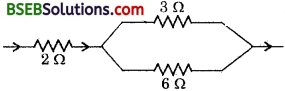3Ω and 6Ω resistances are connected in parallel and this combination is connected in series to 2Ω resistor.

(b) For 1Ω resistance :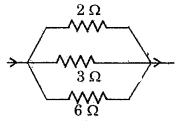All these three resistances are connected in parallel to get equivalent resistance of 1Ω.Question 5.
What is (a) the highest, (b) the lowest total resistance that can be secured by combinations of four coils of resistances 4 Ω, 8 Ω, 12 Ω , 24 Ω ?
(a) For highest resistance, resistors are connected in series:
R = R1 + R2 + R3 + R4
= 4 + 8 + 12 + 24
R = 48 Ω

(b) The lowest resistance will be obtained when these are connected in parallel :Intext Questions (Page 218)

Question 1.
Why does, the cord of an electric heater not glow while the heating element does ?
We know, H = I2Rt
⇒ H ∝ R
The resistance of heating element is very high, so more electrical energy is converted into heat energy, hence it glows. But the cord has very b v resistance, so it does not glow.

Question 2.
Compute the heat generated while transferring 96000 coulombs of charge in one hour through a potential difference of 50 V.
We know, W = QV
⇒ H = QV
= 96500 x 50 Joules = 4825000
= 4.825 x 106Joules
H = 4.825 x 103 Kilojoules

Question 3.
A electrical iron of resistance 20 Ω takes a current of 5 A. Calculate the heat developed in 30 s.
We know, H = I2R£
H = 52 x 20 x 30 .
H = 500.0 Joules
⇒ H = 5 KilojoulesIntext Questions (Page 220)

Question 1.
What determines the rate at which energy is delivered by a current ?
Electrical power determines the rate at which energy is delivered by a current.

Question 2.
An electric motor takes 5 A from a 220 V line. Determine the power of the motor and the energy consumed in 2 h.
Power = VI
P = 220 x 5
P = 1100 Watts
Energy = Power x Time
= 1100 x 2 h x 3600 sec = 3960000 Joule
= 3.96 x 106 Joule = 3.96 x 103 Kilojoule⇒ I1 = 2I2
Hence, current will also become half of its initial value.

### Bihar Board Class 10 Science Chapter 12 Electricity Textbook Questions and Answers

Question 1.
A piece of wire of resistance R is cut into five equal parts. These parts are then connected in parallel. If the equivalent resistance of this combination is R’, then the ratio R/R’ is –
(a) $$\frac {1}{25}$$
(b) $$\frac {1}{5}$$‘
(c) 5
(d) 25
(d) 25

Question 2.
Which of the following forms does not represent electrical power in the circuit ?
(a) I2R
(b) IR2
(c) VI
(d) V2/R
(b) IR2Question 3.
An electric bulb is rated 220 V and 100 W. When it is operated on 110 V, the power consumed will be :
(a) 100 W
(b) 75 W
(c) 50 W
(d) 25 W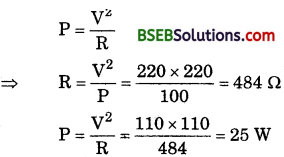(d) 25 W

Question 4.
Two conducting wires of the same material and of equal lengths.and equal diameters are first connected in series and then parallel in a circuit across the same potential differencé. The ratio of heat produced in series and parallel combinations would be –
(a) 1 : 2
(b) 2 : 1
(c) 1 : 4
(d) 4 : 1
Let the resistance of the conducting wire be R Ω.
The equivalent resistance for series combination
R eq = R + R = 2R
The equivalent resistance of parallel combination
R eq = $$\frac{\mathbf{R} \times \mathbf{R}}{\mathbf{R}+\mathbf{R}}=\frac{\mathbf{R}^{2}}{2 \mathbf{R}}=\frac{\mathbf{R}}{2}$$
Let the heat produced be H1 and H2 respectively.(c) H1 : H2 :: 1 :  4

Question 5.
How is a voltmeter connected in the circuit to measure the potential difference between two points ?
Voltmeter is connected in parallel to the appliance across which the potential difference is to be measured.Question 6.
A copper wire has diameter 0.5 mm and resistivity of 1.6 x 10-8 Ω – m. What will be the length of this wire to make its resistance 10 Ω ? How much does the resistance change if the diameter is doubled ?
We know, R = p$$\frac {l}{A}$$
R = Resistance of the conductor
p = resistivity
l = length of the conductor
A = Area of cross-section of conductor
R = 10 Ω and A = πr2Since R ∝$$\frac {1}{A}$$ i.e., R ∝$$\frac{1}{\mathbf{r}^{2}}$$ hence, if diameter is doubled, resistance with the one fourth

Question 7.
The values of current I flowing in a given resistor for the corresponding values of potential difference V across the resistor are given below :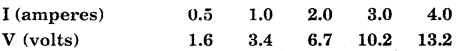Plot a graph between V and I and calculate the resistance of that resistor.
V is kept on y – axis and I on x – axis to plot a graph.Re sistance from graph = $$\frac{\mathrm{V}_{2}-\mathrm{V}_{1}}{\mathrm{I}_{2}-\mathrm{I}_{1}}$$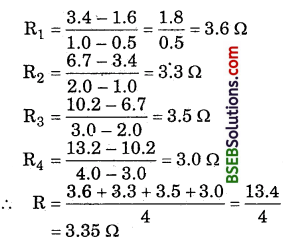Question 8.
When a 12 V battery is connected across an unknown resistor, there is z. Current of 2.5 mA in the circuit. Find the value of the resistance in the resistor.
We know,Question 9.
A battery of 9 V is connected in series with resistors of 0.2 Ω, 0.3 Ω, 0.4 Ω, 0.5 Ω and 12 Ω respectively. How much current would flow through the 12 Ω resistor?
In series combination, same strength of current passes through each and every resistor.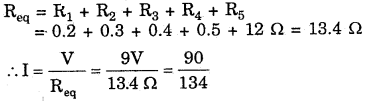I = 0.67 Ampere
∴ Value of I through 12 Ω resistor is 0.67 A.

Question 10.
How many 176 Ω resistors (in parallel) are required to carry 5 A on a 220 V line?
Let the number of resistors = n.n = 4
number of resistors = 4Question 11.
Show how would you connect three resistors, each of resistance 6 Ω, so that the combination has a resistance of (i) 9Ω (ii) 4Ω.
We can connect resistance for (i) 9 Ω as belowThis means that two resistors are connected in parallel, and one is connected in series to these two resistances to get 9Ω resistance.
Again, for 4Ω we will connect them as below :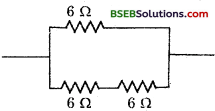Here, two resistances are. connected in series, and this combination is connected to third resistance in parallel to get 4Ω resistance.

Question 12.
Several electric bulbs designed to be used on a 220 V electric supply line, are rated 10 W. How many lamps can be connected in parallel with each other across the two wires of 220 V line if the maximum allowable current is 5 A ?
Resistance of each bulb = R
∴ R = $$\frac{\mathrm{V}^{2}}{\mathrm{P}}$$ (P = power)
= $$\frac{220 \times 220}{10}$$
R = 4840 Ω

Let ‘n’ such bulbs be coniwcted in parallel to 220 V to obtain maximum 5 A current.∴ Number of electric bulbs = 110

Question 13.
A hot plate of electric oven connected to a 220 V line has two resistance coils A and B, each of 24 Ω resistance, which may be used separat4ly, in series, or in parallel. What are the currents in the three cases?
Case (i) : When the plates are connected in series.
∴ R = 24 + 24 = 48 Ω.
∴ I = $$\frac {V}{R}$$ = $$\frac {220}{48}$$ Ampere 4.6 A (approx.)

Case (ii) : When the plates are connected in parallel.
R = $$\frac{24 \times 24}{24+24}=\frac{24 \times 24}{48}$$ = 12Ω
∴ I = $$\frac {V}{R}$$ = $$\frac {220}{12}$$ = 18.3 A (approx.)

Case (iii) : When only one resistance is connected.
I = $$\frac {V}{R}$$ = $$\frac {220}{24}$$ = 9.2 AQuestion 14.
Compare the power used in the 2 Ω resistor in each of the following circuits : (i) a 6 V battery in series with 1 Ω and 2 Ω resistors, and (ii) a 4 V battery in parallel with 12 Ω and 2 Ω resistors.
Case (i)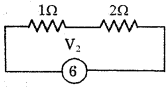V = 6 V, R = 1 + 2 = 3Ω
I = $$\frac {V}{R}$$ = $$\frac {6}{3}$$
I = 2A
p 1 = VI = 6 x 2 = 12 W

Case (ii)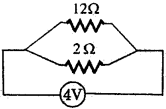V = 4 V (in parallel V is same for both resistors)
R = 2 Ω
∴ I = $$\frac {V}{R}$$ = = $$\frac {4}{2}$$ = 2A
∴ P2 = VI = 4 x 2 = 8 W
$$\frac{P_{1}}{P_{2}}=\frac{12 \mathrm{~W}}{8 \mathrm{~W}}$$
P1 : P2 :: 3 : 2

Question 15.
Two lamps, one rated loo W at 220 V, and the other 60 W at 220 V, are connected in parallel to electric main supply. What current is drawn from the line if the supply voltage is 220 V?
Resistance of first lamp = R1
Resistance of second lamp = R2
We know,When R1 and R2 are connected in parallel,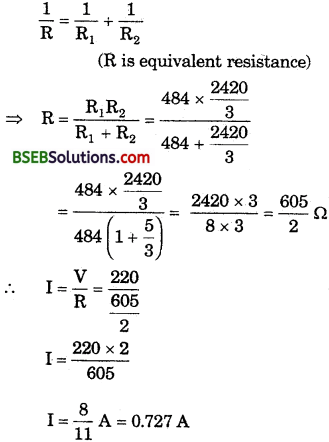Question 16.
Which uses more energy, a 250 W TV set in 1 hr or a 1200 W toaster in 10 minutes ?
Energy = Power x Time
For TV set :
E1 = 250 W x 1 x 3600 sec = 900000 J = 9 x 105 J

For toaster :
E2 = PT = 1200 W x 10 x 60 sec = 720000 = 7.2 x 105 J
⇒ E1 > E2
∴ TV set uses more energy.Question 17.
An electric heater of resistance 8 Ω draws 15 A from the series mains in 2 hours. Calculate the rate at which heat is developed in the heater.
Rate of heat = Power
p = $$\frac {E}{t}$$ = $$\frac{\mathrm{I}^{2} \mathrm{R} t}{t}$$
P = I2R = 152 x 8 = 225 x 8 = 1800 Watt
So heat is developed at the rate of 1800 J/sec.

Question 18.
Explain the following :
(а) Why is the tungsten used almost exclusively for filament of electric lamps ?
(b) Why are the conductors of electric heating devices, such as bread-toasters and electric irons, made of an alloy rather than a pure metal ?
(c) Why is the series arrangement not used for domestic circuits ?
(d) How does the resistance of a wire vary with its area of cross-section ?
(e) Why are copper and aluminium wires usually employed for electricity transmission ?
(a) Tungsten has high melting point and high resistance. So it is used to prepare filament of electric bulbs. Due to high resistance of tungsten, large quantity of heat is produced due to which the bulb glows and due to its high melting point, it does not melt even at higher temperature.
(b) An alloy has the following characteristics :

• It has a high melting point.
• It has high resistance.
• It has high density.

Due to high resistance, it is used to produce large heat in electric heating devices.
H = I2Rt

(c) When appliances are connected in series, the value of equivalent resistance will be very large, as
Req = R1 + R2 + R3 + R4

Due to high value of equivalent resistance, large quantity of heat will be produced in domestic circuit, which may cause fire in the house. But the equivalent resistance for parallel combinations is very low. This is why, series arrangement is not used for domestic circuit.

(d) We know, R = p$$\frac {l}{A}$$
where, R = Resistance of wire, p = resistivity of wire, l = length of the wire, A = area of cross-section.
∴ R ∝ $$\frac {1}{A}$$
⇒ R ∝$$\frac{1}{\pi r^{2}}$$
∴ R ∝ $$\frac{1}{r^{2}}$$

Resistance of conductor increases as radius of cross¬sectional area decreases and vice-versa.

(e) Silver, copper and aluminium are the best conductors of electricity. Since silver is too costly, so copper and aluminium are usually employed for electricity transmission.### Bihar Board Class 10 Science Chapter 12 Electricity Textbook Activities

Activity 12.1 (Page 203)

Questions Based on the Activity

Question 1.
What is nichrome ?
Nichrome is an alloy of nickel, chromium, manganese and iron metals.’

Question 2.
What is a voltmeter?
Voltmeter is a device to measure the potential difference between two points.

Question 3.
What is an ammeter?
Ammeter is a device to measure the strength of electric current in an electric circuit.

Question 4.
What is cell ?
Cell is a device to maintain the potential difference between the ends of a conductor for a long time.

Question 5.
What does straight line graph between V vs. I represent ?
The straight line graph represents that the strength of current in a conductor varies directly with potential difference i.e.,
V ∝ IQuestion 6.
What is the name of physical quantity which is equal to V/I ?
Y = R, where R is called the resistance of the conductor.

Activity 12.2 (Page 205)

Questions Based on the Activity

Question 1.
When four cells of 1.5 V are connected in series, how much resultant potential difference will be developed?
1.5 x 4 = 6.0
So, 6 volt potential difference will be developed.

Question 2.
A torch bulb is marked as 10 W and 3.0 V. What does this refer ?
It refers that the bulb will consume energy at a rate of 10 watts when it is operated at the potential difference of 3.0 volt.

Question 3.
Why does the reading of an ammeter vary when the bulb and the nichrome wire are kept respectively in the gap?
It is because the resistances of nichrome and bulb are different and we know that V oc I. So there is a variation in the reading of the ammeter.Activity 12.3 (Page 206)

• Complete an electric circuit consisting of a cell, an ammeter, a nichrome wire of length L [say, marked (1)] and a plug key as shown in Fig. 12.9.Fig. 12.9. Electric circuit to study the factors on which the resistance of conducting wires depends.
• Now, plug the key. Note the current in the ammeter.
• Replace the nichrome wire by another nichrome wire of same thickness but twice the length, that is 2L [marked (2) in the Fig. 12.9].
• Now replace the wire by a thicker niehrome wire, of the same length L [marked (3)3. A thicker wire has a larger cross sectional area. Again note down the current through the circuit. ,
• Instead of taking a niehrome wire, connect a copper wire [marked (4) in Fig. 12.9] in the circuit. Let the wire be of the same length and same area of cross-section as that of the first niehrome wire [marked (1)]. Note the value of the current.
Notice the difference in the current in all cases.

Question 1.
Does the current depend on the length of the conductor?
Yes.

Question 2.
Does the current depend on the area of cross¬section of the wire used?
Yes.

Question 3.
What happens to resistance when length of conductor is doubled without affecting the thickness of conductor ?
The resistance of conductor will become double.

Question 4.
What happens to resistance if the radius of cross¬section is halved without changing the length of conductor ?
The resistance of conductor becomes 4 times that of the original resistance.Question 5.
Why does the resistance of aluminium and iron rods of same size and same thickness not equal ?
Since the resistance of conductor depends uport the nature of conductor, so the resistances of both rods are different. .

Activity 12.4 (Page 210)

Questions Based on the Activity

Question 1.
What is series combination of resistances ?
The end to end connection of resistances in an electric circuit is called series combination of resistances.

Question 2.
Which factor of electric circuit remains same for each resistance when connected in series?
The strength of current through each resistance remains the same when resistances are connected in series.

Activity 12.5 (Page 211)

Questions Based on the Activity

Question 1.
Is potential difference same between ends of each resistance connected in series ?
No, potential difference between ends of different resistances connected in series is not the same.

Question 2.
If r1 and r2 resistances are connected in series and following observations are made :
(a) potential difference between ends of r1 = V1
(b) potential difference between ends of r1 = V2
(c) potential difference between r1 and r2 = V
Then what is relation among V1, V2 and V ?
V = V1 + V2Activity 12.6 (Page 213)

• Make a parallel combination, XY, of three resistors having resistances R1 R2, and R3, respectively. Connect it with a battery, a plug key and an ammeter as shown in –
• Fig. 12.16. Also connect a voltmeter in parallel with the combination of resistors.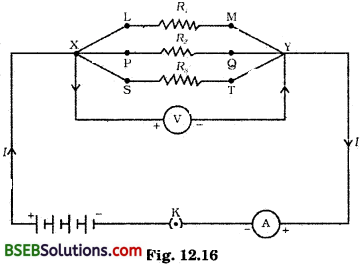• Plug the key and note the ammeter reading. Let the current be I. Also take the voltmeter reading. It gives the potential v difference V across the combination. The potential difference across each resistor is also V. This can be checked by connecting the voltmeter across each individual resistor (see Fig. 12.17).
• Take out the plug from the key. Remove the ammeter and voltmeter from the circuit. Insert the ammeter in series with the resistor Rx as shown in Fig. 12.17. Note the ammeter reading, I1.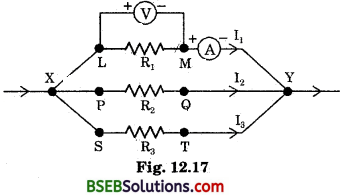• Similarly, measure the currents through R2 and R3. Let these be I2 and I3, respectively.

Questions Based on the Activity

Question 1.
What is parallel combination of resistances ?
When the ends of conductors are kept between two points taking together the ends of conductor in an electric circuit, it is called
parallel combination of resistances.

Question 2.
Which factor of electric circuit remains same for each resistances when connected in parallel ?
The potential difference is the same between ends of resistances when they are connected in parallel.Question 3.
R1 and R2 are connected in parallel and I1 and I2 are respectively the strength of current through Rx and R2. Then what will be the total strength of current in the electric circuit ?
If I be the total strength of electric current in the electric circuit, then
I = l1 + I2

### Bihar Board Class 10 Science Chapter 12 Electricity NCERT Exemplar Problems

Question 1.
A child has drawn the electric circuit to Study Ohm’s law as shown in the figure given below. His teacher told that the circuit diagram needs correction. Study the circuit diagram and redraw it after making all corrections.Question 2.
Three 2 Q resistors, A, B and C, are connected as shown in the figure given below. Each of them dissipates energy and can withstand a maximum power of 18 W without melting. Find the maximum current that can flow through the three resistors.Maximum current through resistor A = $$\sqrt{\frac{18}{6}}$$ A = 3A
Thus, the maximum current through resistors B and C each :
3 x $${\frac{1}{2}}$$ A = 1.5 AQuestrion 3.
Should the resistance of an ammeter be low or high? Give reason.
It should be as close to zero as possible. Ideally it . should be zero ohm. If it is non-zero and substantial it will affect the true current.

Question 4.
Draw a circuit diagram of an electric circuit containing a cell, a key, an ammeter, a resistor of 2 Ω in series with a combination of two resistors (4Ω. each) in parallel and a voltmeter across the parallel combination. Will the potential difference across the 2 Ω resistor be the same as that across the parallel combination of 4 Ω resistors? Give reason.
Yes. Total resistance of the parallel combination is also 2 ohm (2 Ω) because when two resistances connected in parallel,resultant resistance is given by –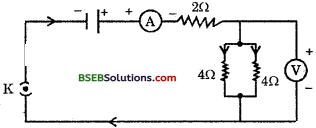Question 5.
How does use of a fuse wire protect electrical appliances?
If a current larger than a specified value flows in a circuit, temperature of fuse wire increases to its melting point. The fuse wire melts and the circuit breaks. The flow of current stops in the circuit and electrical appliances are protected.
difference across the lamp will take place? Give reason.
Here I = 1 A
V = 10 V .
Let the resistance of the electrical lamp = R1
The resistance of conductor (R2) = 5Ω
∴ By Ohm’s Law
V = I R
10 = 1 (R1 + R2)
[ ∴ In a series combination, total resistance of the circuit is the algebraic sum of resistances in the circuit] .
10 = 1 (R1 + 5)
R1 = 10 – 5 = 5 Ω
Now, if a resistance of 10 Ω is connected in parallel with this series combination, then total resistance of the circuit would be
$$\frac{1}{R}=\frac{1}{10}+\frac{1}{10}=\frac{1}{5}$$
R = 5 Ω
Thus, there Would be no change in current flowing through 5 Ω conductor. Also there would be no change in the potential difference across the lamp.Question 9.
Why is parallel arrangement used in domestic wiring?
A parallel circuit divides the current through the electrical gadgets. The total resistance in a parallel circuit is decreased as per equation :
$$\frac{1}{R_{p}}=\frac{1}{R_{1}}+\frac{1}{R_{2}}+\frac{1}{R_{3}}$$
This is helpful particularly when each gadget has different resistance and requires different current to operate properly.
So, a parallel arrangement is used in domestic wiring.

Question 10.
B1, B2 and Bg are three identical bulbs connected as shown in the given figure. When all the three bulbs glow, a current of 3 A is recorded by the ammeter A.
(i) What happens to the glow of the other two bulbs when the bulb Bt gets fused ?
(ii) What happens to the reading of A1, A2, A3 and A when the bulb B2 gets fused ?
(iii) How much power is dissipated in the circuit when all the three bulbs glow together ?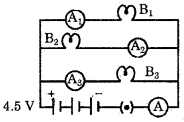(i) The glow of the bulbs B2 and Bg will remain the same.
(ii) A1 shows 1 ampere, A2 shows zero, A3 shows I ampere and A shows 2 ampere.
(iii) P = V x I = 4.5 x 3 = 13.5 W

Question 11.
Does Ohm’s law hold gopd under all conditions? Comment.

• Ohm’s law does not hold good if
• current is continuously passed through the circuit for a longtime.
• temperature varies drastically as resistance offered by different materials change.
• resistors or circuits are of micro/nano level.

Question 12.
Three incandescent bulbs of 100 W each are connected in series in an electric circuit. In another circuit another set of three bulbs of the same wattage are connected in parallel to the same source.
(a) Will the bulb in the two circuits glow with the same brightness? Justify your answer.
(b) Now let one bulb in both the circuits get fused. Will the rest of the bulbs continue to glow in each circuit? Give reason.
(a) No. The resistance of the bulbs in series will be three times the resistance of single bulb. Therefore, the current in the series combination will be one-third compared to current in each bulb in parallel combination. The parallel combination of bulbs will glow more brightly.(b) The bulbs in series combination will stop glowing as the circuit is broken and current is zero. However, the bulbs that are not fused in parallel combination shall continue to glow with the same brightness.

Question 13.
What is electrical resistivity of a material? What is it’s unit?
Describe an experiment to study the factors on which the resistance of conducting wire depends.
Resistivity is numerically equal to the resistance of a wire of unit length having an unit area of cross-section. Its unit is ohm metre (Ω m).Factors on which the resistance of conducting wire depends: Connect a circuit consisting of a cell, an ammeter, a wire of a material of high resistance, for example, nichrome of length l (marked 1 in the figure) and a key as shown in figure. Insert the key anu .»ote the current in the ammeter. Replace the wire by another one having the same thickness but the length 21 (marked 2 in figure). We will note that the current has now decreased to one half.

Now, if we take a thicker nichrome wire, i.e., of a larger cross-sectional area but having the same length l (marked 3), we will find that the current in the ammeter has increased this time, indicating thereby that the flow of current depends on the cross-sectional area of the wire.

Now, instead of taking a nichrome wire, if we take a wire of another material having the same length and the same cross-sectional area as that of the nichrome wire (marked 4), we will find that the current in the ammeter has changed. This shows that the flow of the current from the same source also depends on the material of the wire.

Thus, it is clear that the resistance of the conductor depends on (i) its length, (ii) its area of cross-section, and (Hi) the nature of its material. Precise measurements have shown that resistance of a uniform metallic conductor is directly proportional to its length (Z) and inversely proportional to the area of cross-section (A), i.e.;
R ∝ $$\frac {l}{A}$$Question 14.
How will you infer with the help of an experiment that the same current flows through every part of the circuit, containing three resistances in series connected to a battery?
Join three resistors of different values in series. Connect them with a battery, an ammeter and a plug key as shown in the fig. The resistors of values like 1Ω, 2Ω, 3Ω, etc. and a battery of 6 V can be used for performing this activity. Plug the key and note the ammeter reading. Change the position of ammeter to anywhere in between the resistors. Note the ammeter reading each time.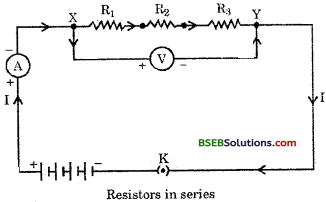It is observed that the value of the current in the ammeter is the same, independent of its position in the electric circuit.

Question 15.
How will you conclude that the same potential difference (voltage) exists across three resistors connected in a parallel arrangement to a battery?
Make a parallel combination, XY, of three resistors having resistances R^, R„ and R3 respectively. Connect it with a battery, a plug key and-an ammeter as shown in the figure. Also connect a voltmeter in parallel with the combination of resistors. Plug the key and note the ammeter reading. Let the current be I. Also take the voltmeter reading. It gives the potential difference V, across the combination. The potential difference across each resistor is also V. This can be checked by connecting the voltmeter across each individual resistor.Thus, it can be concluded that the same potential difference (voltage) exists across three resistors connected in a parallel arrangement to a battery.

Question 16.
What is Joule’s heating effect? How can it be demonstrated experimentally? List its four applications in daily life.
Whenever a source supplies energy in a circuit, to maintain the current, the source has to keep expending its energy. A part of the source energy in maintaining the current may be consumed into useful work while the rest of the source energy may be expended in heat to raise the temperature of gadget. This is known as heating effect of electric current. This heat generated is equal to the product of square of current passing through the circuit, resistance in the circuit and time for which current is flowing in the circuit, i.e;
H = I2Rt ,
This is Joule’s heating effect.Experimental demonstration of Joule’s law of heating:
Consider a current I flowing through a resistor of resistance R. Let the potential difference across it be V. Let t be the time during which a charge Q flows across. The work done in moving the charge Q through a potential difference V is VQ. Therefore, the source must supply energy equal to VQ in time t. Hence the power input to the circuit by the source is
P = V $$\frac {Q}{t}$$ = VI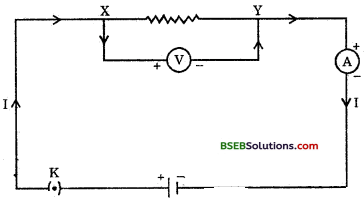Or the energy supplied to the circuit by the source in time t is P x t, that is Vh. The energy expended by the source gets dissipatedin the resistor as heat. Thus, for a steady current I, ; the amount of heat H produced in time t is
H = VIt
Applying Ohm’s law, we get
H = I2Rt
This is known as Joule’s law of heating.
Joule’s heating effect is utilised in devices such as electric heater, electric iron, electric oven, bulb, toaster, kettle, etc.

Question 17.
Find out the following in the electric circuit given in the figure.
(a) Effective resistance of two 8 Ω resistors in the combination
(b) Current flowing through 4Ω resistor
(c) Potential difference across 4Ω resistance
(d) Power dissipated in 4Ω resistor
(e) Difference in ammeter readings, if any.